In this post I’ll work with this dataset from Kaggle which is related to the number of suicides in several countries across many years. However, I won’t make any kind of inferential analysis about the data. My main goal is to make a tutorial about how to work with factors in R by showing the powerful tidyverse package called `forcats`. I will explore some variables that can be turned into factors and show you the main functions of `forcats` to help you wrangle data.

Now, we can load our libraries.

``````# load libraries
library(tidyverse) # wrangling and data visualization - includes the forcats package
library(here) # sets a path to your file
library(kableExtra) # visualize html tables
library(scales) # creates different types of scales(e.g. commas, dollar sign, etc...)
library(viridis) # colour palette
library(ggdark) # themes for plots
library(hrbrthemes) # add fonts in this case
options(scipen = 999) # remove scientific notation``````

We will now open our dataset. We will call it “suicides”.

``````# open dataset
glimpse(suicides)``````
``````## Observations: 27,820
## Variables: 12
## \$ country              <chr> "Albania", "Albania", "Albania", "Albania...
## \$ year                 <dbl> 1987, 1987, 1987, 1987, 1987, 1987, 1987,...
## \$ sex                  <chr> "male", "male", "female", "male", "male",...
## \$ age                  <chr> "15-24 years", "35-54 years", "15-24 year...
## \$ suicides_no          <dbl> 21, 16, 14, 1, 9, 1, 6, 4, 1, 0, 0, 0, 2,...
## \$ population           <dbl> 312900, 308000, 289700, 21800, 274300, 35...
## \$ `suicides/100k pop`  <dbl> 6.71, 5.19, 4.83, 4.59, 3.28, 2.81, 2.15,...
## \$ `country-year`       <chr> "Albania1987", "Albania1987", "Albania198...
## \$ `HDI for year`       <dbl> NA, NA, NA, NA, NA, NA, NA, NA, NA, NA, N...
## \$ `gdp_for_year (\$)`   <dbl> 2156624900, 2156624900, 2156624900, 21566...
## \$ `gdp_per_capita (\$)` <dbl> 796, 796, 796, 796, 796, 796, 796, 796, 7...
## \$ generation           <chr> "Generation X", "Silent", "Generation X",...``````
``````# explore dataset
suicides %>%
``````## # A tibble: 6 x 12
##   country  year sex   age   suicides_no population `suicides/100k ~
##   <chr>   <dbl> <chr> <chr>       <dbl>      <dbl>            <dbl>
## 1 Albania  1987 male  15-2~          21     312900             6.71
## 2 Albania  1987 male  35-5~          16     308000             5.19
## 3 Albania  1987 fema~ 15-2~          14     289700             4.83
## 4 Albania  1987 male  75+ ~           1      21800             4.59
## 5 Albania  1987 male  25-3~           9     274300             3.28
## 6 Albania  1987 fema~ 75+ ~           1      35600             2.81
## # ... with 5 more variables: `country-year` <chr>, `HDI for year` <dbl>,
## #   `gdp_for_year (\$)` <dbl>, `gdp_per_capita (\$)` <dbl>, generation <chr>``````

The age variable seems to be an interesting one to use in this tutorial. We can turn it into a factor variable with the `as.factor` function.

``````# lets work with the age variable. Let's turn age into a factor variable
suicides_tbl <- suicides %>%
mutate(age = as.factor(age))``````

### fct_relevel

Now that we have the age variable as factor we can check its levels.

``````# check levels of the variable age
levels(suicides_tbl\$age)``````
``````##  "15-24 years" "25-34 years" "35-54 years" "5-14 years"  "55-74 years"
##  "75+ years"``````

As we can see the levels of the factor variable age are not in the correct order. The level “5-14 years” appears in the 4th position, when it should be in the first one. To order the levels in the right way , we should use the `fct_relevel` function. We can do this by writing all arguments of the function in the correct order:

``````# forcats functions
# 1: fct_relevel()
suicides_tbl <- suicides_tbl %>%
mutate(age = fct_relevel(age,
"5-14 years",
"15-24 years",
"25-34 years",
"35-54 years",
"55-74 years",
"75+ years"
))

suicides_tbl %>%

pull(age) %>%

levels()``````
``````##  "5-14 years"  "15-24 years" "25-34 years" "35-54 years" "55-74 years"
##  "75+ years"``````

Or we can use the argument `after` which in this case will correspond to 0, because we want the level `5-14 years` to be the first one.

``````# fct_relevel alternative
suicides_tbl %>%
mutate(age = fct_relevel(age,
"5-14 years", after = 0
)) %>%
pull(age) %>%
levels()``````
``````##  "5-14 years"  "15-24 years" "25-34 years" "35-54 years" "55-74 years"
##  "75+ years"``````

### fct_reorder

One interesting function in `forcats` is `fct_reorder`. As you can see in the plot below, the age variable is ordered based on its levels.

``````# make a plot without fct_reorder
suicides_tbl %>%
filter(year== 2015,
country == "United Kingdom") %>%
group_by(age) %>%
summarise(suicides_total = sum(suicides_no)) %>%
mutate(prop = suicides_total / sum(suicides_total))%>%
ggplot(aes(age, suicides_total, fill = suicides_total,
color = NULL)) +
geom_col() +
scale_fill_viridis(option = "inferno", direction = -1) +
labs(title = "Suicides in the UK", subtitle = "Year 2015", y = "Total Number of Suicides",
x = "Age", fill = "Number of Suicides") +
scale_y_continuous(labels = comma,
expand = c(0,0)) +
coord_flip() +
theme_minimal() +
theme(plot.title = element_text(family = "Agency FB", face = "bold",
size = 25, hjust = .5),
plot.subtitle = element_text(family = "Agency FB", face = "bold",
size = 15),
axis.title = element_text(family = "Agency FB", size = 15),
axis.text = element_text(family = "Agency FB", size = 15),
legend.text = element_text(family = "Agency FB", size = 15),
legend.title = element_text(family = "Agency FB", size = 15))``````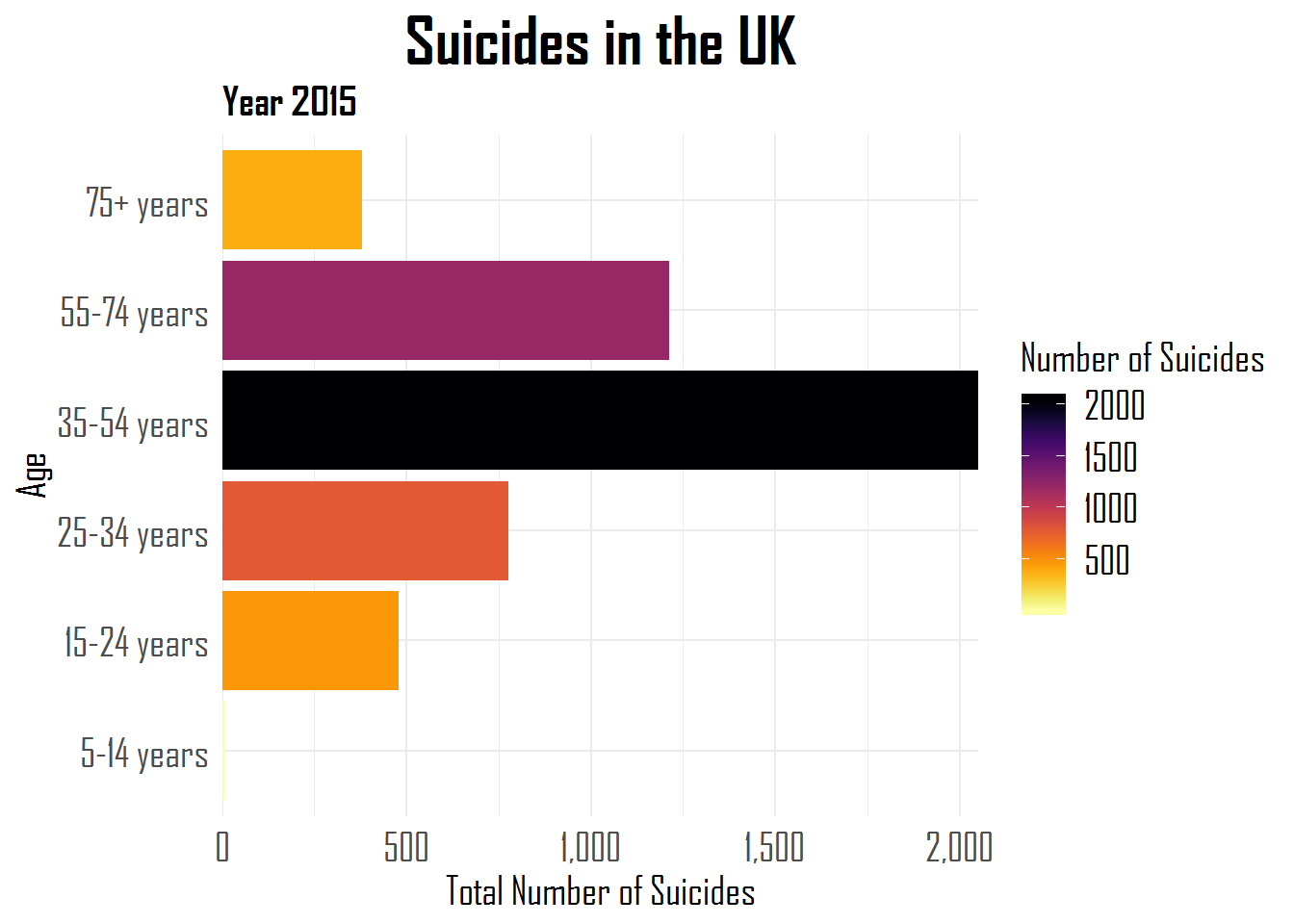However, in some cases we want the factor variables reordered in plots based on the values of some continuous variable. With that in mind we can use `fct_reorder` to reorder the age variable based on the total number of suicides.

``````# forcats
# 2: fct_reorder()
suicides_tbl %>%
filter(year== 2015,
country == "United Kingdom") %>%
group_by(age) %>%
summarise(suicides_total = sum(suicides_no)) %>%
mutate(prop = suicides_total / sum(suicides_total))%>%
ggplot(aes(fct_reorder(age, suicides_total), suicides_total, fill = suicides_total,
color = NULL)) +
geom_col() +
scale_fill_viridis(option = "inferno", direction = -1) +
labs(title = "Suicides in the UK", subtitle = "Year 2015", y = "Total Number of Suicides",x = "Age", fill = "Number of Suicides") +
scale_y_continuous(labels = comma,
expand = c(0,0)) +
coord_flip() +
theme_minimal() +
theme(plot.title = element_text(family = "Agency FB", face = "bold",
size = 25, hjust = .5),
plot.subtitle = element_text(family = "Agency FB", face = "bold",
size = 15),
axis.title = element_text(family = "Agency FB", size = 15),
axis.text = element_text(family = "Agency FB", size = 15),
legend.text = element_text(family = "Agency FB", size = 15),
legend.title = element_text(family = "Agency FB", size = 15))``````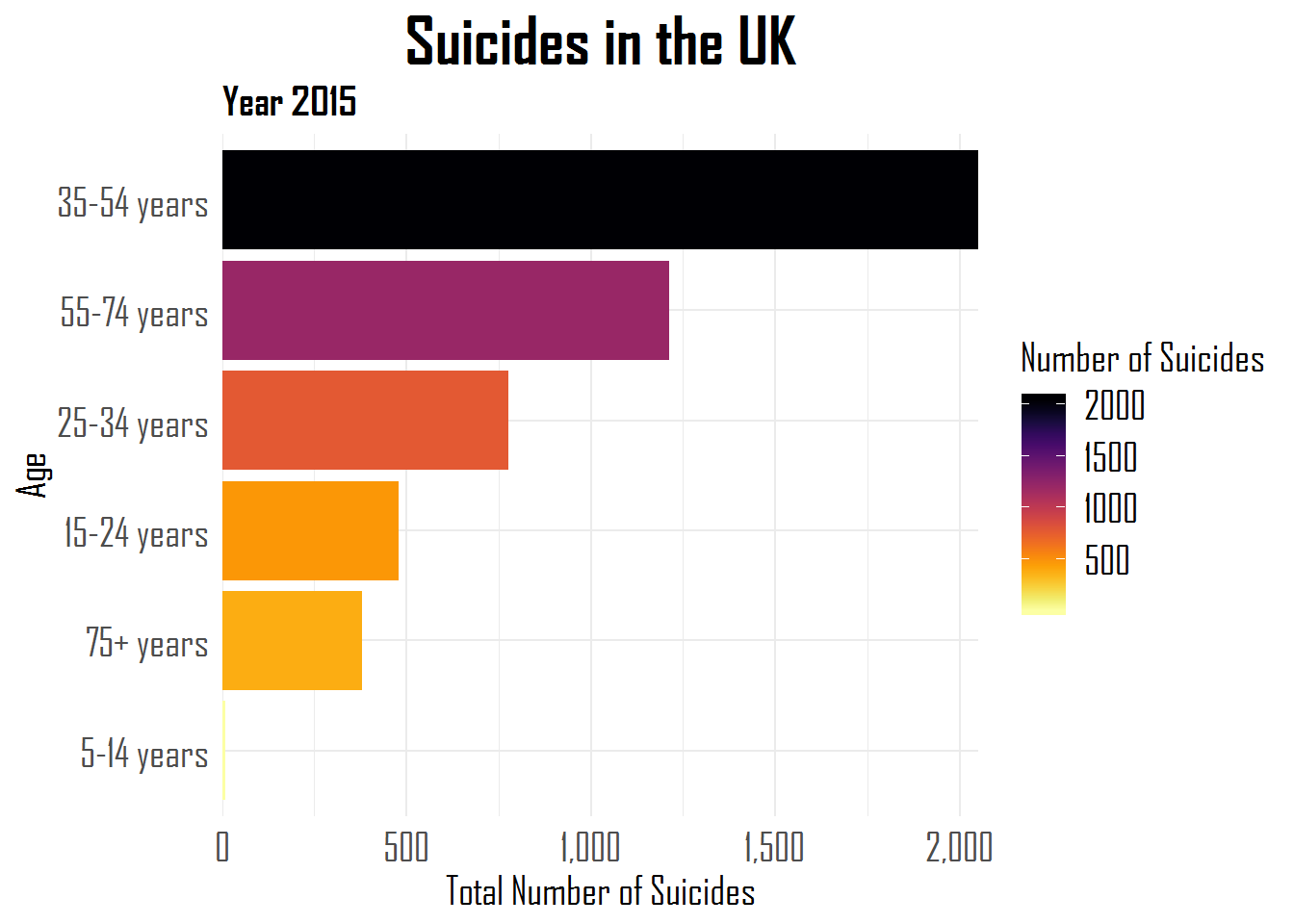This plot looks clearer than the last one. The age variable is reordered according to the number of suicides.

### fct_explicit_na

Let’s work now with another variable of the dataset. We will create 5 levels of Human Development Index based on the variable `HDI for year`. We will turn this variable into a factor and relevel it again with `fct_relevel` and check for missing values.

``````suicides_tbl <- suicides_tbl %>%
mutate(hdi_cat = case_when(`HDI for year` >= 0.80 ~ "Very High Development",
`HDI for year` >= 0.70 ~ "High Development",
`HDI for year` >= 0.55 ~ "Medium Development",
`HDI for year` >= 0.35 ~ "Low Development",
`HDI for year` < 0.35 ~ "Very Low Development"
) %>% as.factor) %>%
mutate(hdi_cat = fct_relevel(hdi_cat,
"High Development", after = 2))

# check missing values
any(is.na(suicides_tbl\$hdi_cat))``````
``##  TRUE``

Given that we have missing values, we can use the function `fct_explicit_na` to give it a name.

``````# forcats
# 3: fct_explicit_na()
suicides_tbl <- suicides_tbl %>%
mutate(hdi_cat = fct_explicit_na(hdi_cat, na_level = "Missing"))``````

### fct_lump

We can also lump levels together in `forcats` with `fct_lump`. For instance, we have 4 levels within the variable correspondent to the categories of Human Development Index (hdi_cat), though we may be interested in lumping together the least common factors into a unique level. We can rename this new level with the argument `other_level`. We can lump according to two arguments, `n =` being the first one shown:

``````# forcats
# 4: fct_lump to lump levels
# n argument
suicides_tbl %>%
na.omit() %>%
mutate(hdi_lumped = fct_lump(hdi_cat, n = 2, other_level = "Average/Low Development")) %>%
count(hdi_lumped) %>%
mutate(prop = n / sum(n)) %>%
arrange(desc(n))``````
``````## # A tibble: 3 x 3
##   hdi_lumped                  n  prop
##   <fct>                   <int> <dbl>
## 1 Very High Development    3600 0.430
## 2 High Development         2952 0.353
## 3 Average/Low Development  1812 0.217``````

In this case we retained the two most common categories of Human Development Index present in our dataset, while the remaining categories were put together into the variable “Average/Low Development”.

The second argument to lump together is called `prop`. This argument considers the proportions of each level. As you can see with the `prop = 0.20` all levels with less than 20% will be grouped along. In this case, we have 3 unique HDI categories and one that corresponds to the `other_level`.

``````# prop argument
suicides_tbl %>%
na.omit() %>%
mutate(hdi_relevel = fct_lump(hdi_cat, prop =0.20, other_level = "Below Average")) %>%
count(hdi_relevel) %>%
mutate(prop = n / sum(n))``````
``````## # A tibble: 4 x 3
##   hdi_relevel               n    prop
##   <fct>                 <int>   <dbl>
## 1 Medium Development     1752 0.209
## 2 High Development       2952 0.353
## 3 Very High Development  3600 0.430
## 4 Below Average            60 0.00717``````

### fct_infreq

Moving on to other functions in `forcats`, another interesting one is `fct_infreq`, combined with `ggplot2`. This function is pretty neat when you want to reorder factor levels by frequency.

``````# forcats
# 5: fct_infreq
suicides_tbl %>%
na.omit() %>%
ggplot(aes(fct_infreq(hdi_cat))) +
geom_bar(stat = "count") +
labs(x = "HDI Level", y = "Count") +
theme_minimal() ``````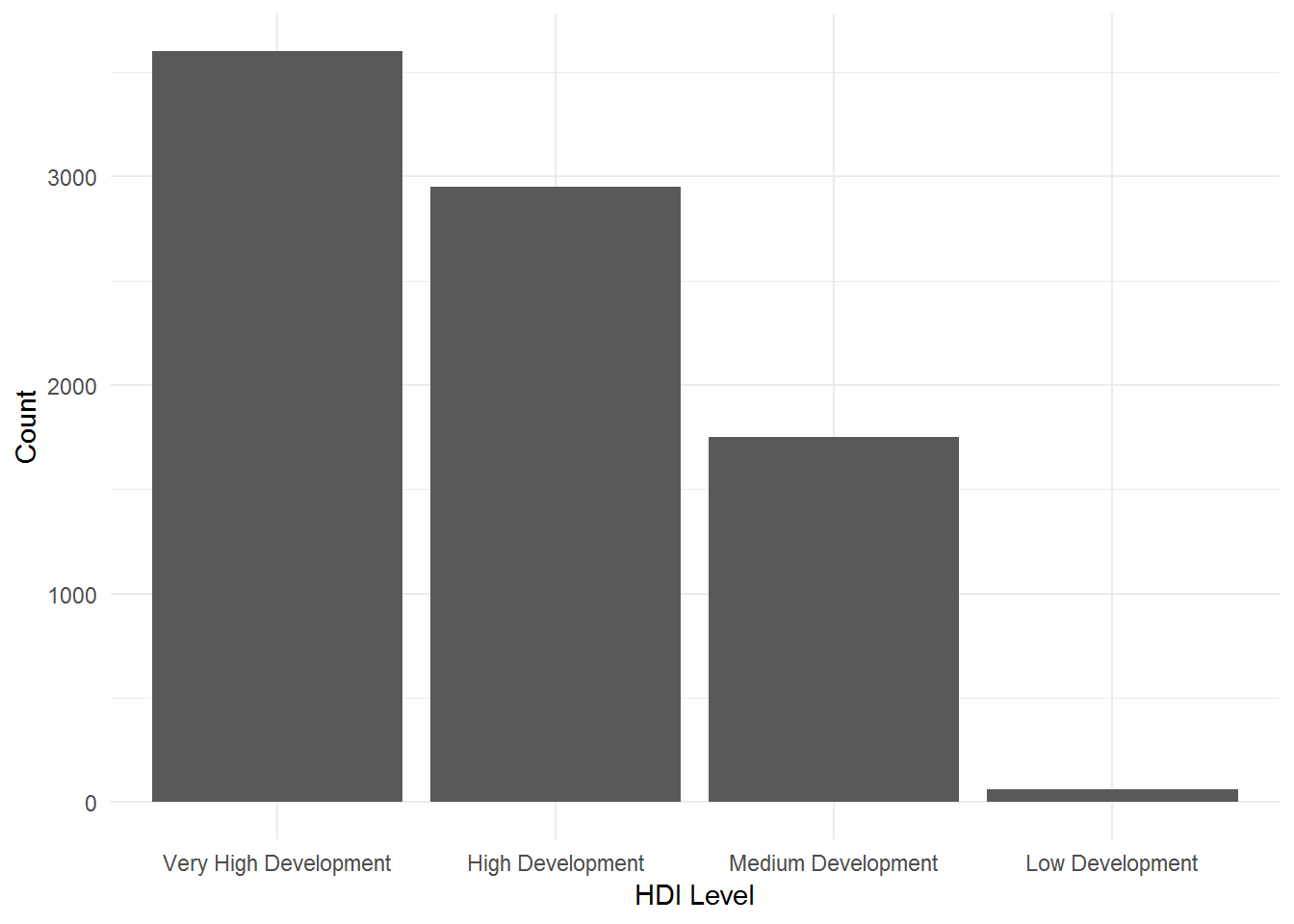### fct_rev

However, we still have an order from right (more cases) to left (less cases). If you want to reorder with the terms of frequency from left (less cases) to right (more cases) you have to use `fct_rev` along with `fct_infreq`.

``````# forcats
# 6: fct_rev
suicides_tbl %>%
na.omit() %>%
ggplot(aes(fct_rev(fct_infreq(hdi_cat)))) +
geom_bar(stat = "count") +
labs(x = "HDI Level", y = "Count") +
theme_minimal() ``````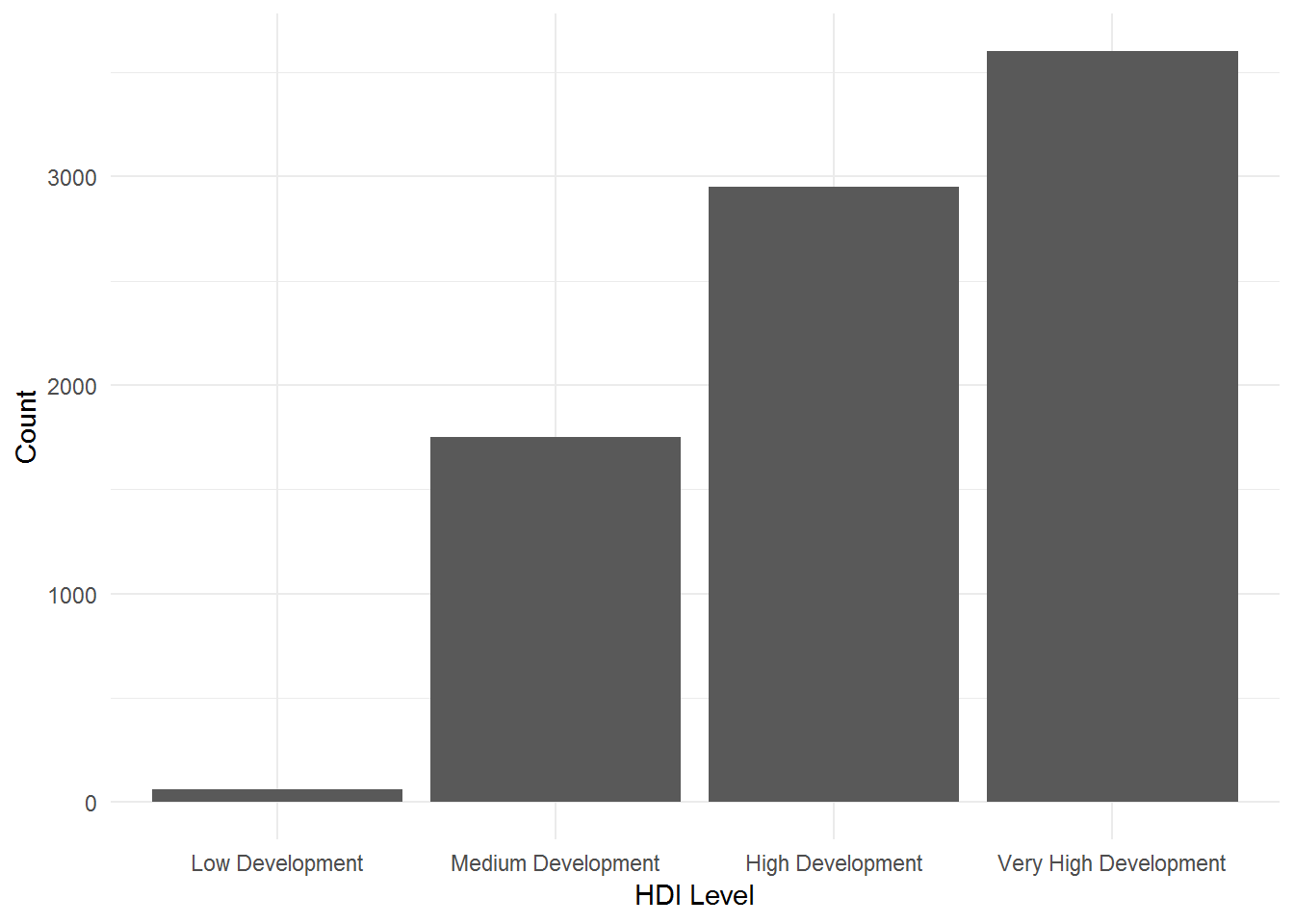### fct_count

If we would be interested in counting the cases in each level, we could simply use `fct_count`.

``````# forcats
# 7: fct_count()
fct_count(suicides_tbl\$hdi_cat)``````
``````## # A tibble: 5 x 2
##   f                         n
##   <fct>                 <int>
## 1 Low Development          60
## 2 Medium Development     1752
## 3 High Development       2952
## 4 Very High Development  3600
## 5 Missing               19456``````

### fct_unique

Or to know the unique levels within our factors, we could use `fct_unique`.

``````# forcats
# 8: fct_unique()
fct_unique(suicides_tbl\$hdi_cat) ``````
``````##  Low Development       Medium Development    High Development
##  Very High Development Missing
## 5 Levels: Low Development Medium Development ... Missing``````

### fct_collapse

Now, let’s work with another variable from our dataset. The variable “generation” has 6 levels: “Silent”, “G.I. Generation”, “Boomers”, “Generation X”, “Generation Z”, and “Millennials”. We could be interested in grouping together some of the levels in a unique one. For this we can use the function `fct_collapse`.

``````# forcats
# 9: fct_collapse()
suicides_tbl %>%
mutate(generation = as.factor(generation)) %>%
mutate(generations = fct_collapse(generation,
"Older Generations" = c("Silent", "G.I. Generation", "Boomers"),
"Younger Generations" = c("Generation X", "Generation Z", "Millenials")
)) %>%
pull(generations) %>%
levels()``````
``##  "Older Generations"   "Younger Generations"``

### fct_other

There might be some cases, where you want to compare one or more levels of a factor variable against all the other levels. For that, you can use the `fct_other`. Let’s imagine that you wanted to create a new factor variable where you wanted to compare the level “Silent” generation against all the other generation levels. In this case, we could use `fct_other` and the argument `keep` equals “Silent”.

``````# forcats
# 10: fct_other()
suicides_tbl %>%
mutate(silent_against_other = fct_other(generation, keep = "Silent")) %>%
pull(silent_against_other) %>%
levels()``````
``##  "Silent" "Other"``

### fct_recode

There are other cases, where you just want to recode your factor variables. In such cases, `fct_recode` is of great help.

``````# forcats
# 11: fct_recode()
suicides_tbl %>%
mutate(age_levels = fct_recode(age,
"Child" = "5-14 years",
"Senior" = "75+ years")) %>%
pull(age_levels) %>%
levels()``````
``````##  "Child"                  "Adolescent/Young Adult"

### fct_reorder2

When you intend to map colors in a line plot where your goal is to reorder a value according to values more associated with the y variable, `fct_reorder2` is of great use. In this example below, we are reordering the lines’ colors according to the higher total number of suicides by age group during the nineties in the USA.

``````# forcats
# 12: fct_reorder2()
suicides_tbl%>%
filter(year > 1989, year < 2000, country == "United States") %>%
group_by(year, age) %>%
summarise(suicides_total = sum(suicides_no)) %>%
ggplot(aes(year, suicides_total, colour = fct_reorder2(age, year, suicides_total))) +
geom_line(size = 2.5) +
scale_x_continuous(expand = c(0,0),
breaks = c(seq(1990, 1998, 2)),
labels = c(1990, 1992, 1994, 1996, 1998),
limits = c(1990, 1999))  +
scale_colour_viridis_d(option = "inferno") +
labs(title = "Suicides in the USA", subtitle = "90's decade",
y = "Total Number of Suicides",
x = "Year", colour = "Age") +
theme_minimal() +
theme(plot.title = element_text(family = "Agency FB", face = "bold",
size = 25, hjust = .5),
plot.subtitle = element_text(family = "Agency FB", face = "bold",
size = 15, hjust = 0.5),
axis.title = element_text(family = "Agency FB", size = 15),
axis.text = element_text(family = "Agency FB", size = 15),
legend.text = element_text(family = "Agency FB", size = 15),
legend.title = element_text(family = "Agency FB", size = 15))``````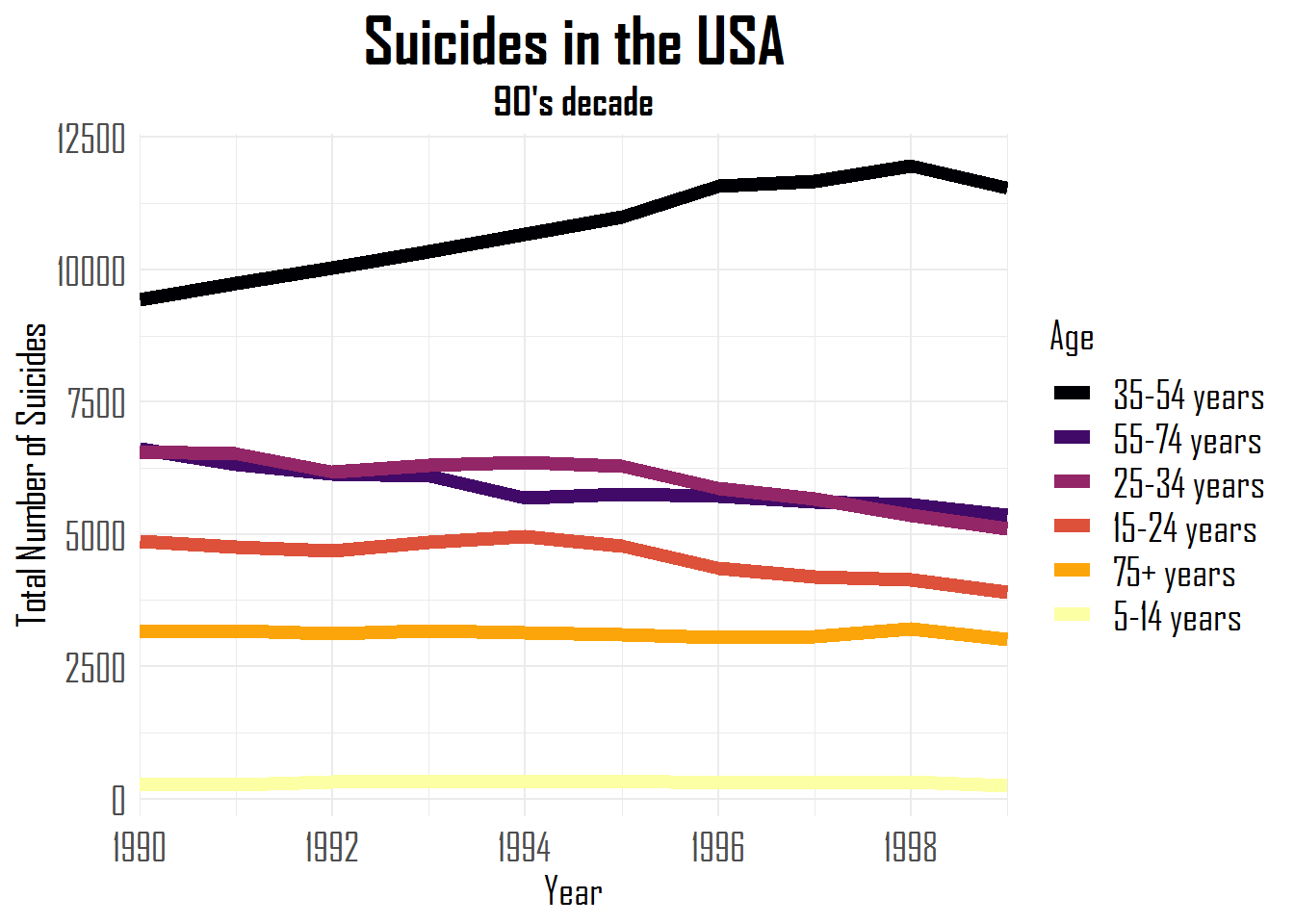### fct_relabel

We can also relabel factor variables with `fct_relabel`. Just to give a quick example, we can remove the word “years” from our age variable.

``````# forcats
# 13: fct_relabel
suicides_tbl\$age %>% fct_relabel(~ str_replace_all(.x, "years", " ")) %>% head()``````
``````##  15-24   35-54   15-24   75+     25-34   75+
## Levels: 5-14   15-24   25-34   35-54   55-74   75+``````

### fct_anon

There might be also some cases where you wish to anonymize your data, then you can use the `fct_anon`.

``````# forcats
# 14: fct_anon
suicides_tbl %>%
mutate(generation = as.factor(generation) %>% fct_anon()) %>%
group_by(generation) %>%
count()``````
``````## # A tibble: 6 x 2
## # Groups:   generation 
##   generation     n
##   <fct>      <int>
## 1 1           2744
## 2 2           1470
## 3 3           4990
## 4 4           5844
## 5 5           6364
## 6 6           6408``````

### fct_shift

When you have a factor whose levels are not in the desired and/ or correct order you can use the `fct_shift` function and add positive (shift to the left) or negative (shift to right) numbers to the `n` argument. We can check it in this example without `fct_shift`:

``````suicides_tbl %>%
mutate(generation = as.factor(hdi_cat)) %>%
pull(generation) %>%
levels()``````
``````##  "Low Development"       "Medium Development"    "High Development"
##  "Very High Development" "Missing"``````

And with `fct_shift` where each level is moved one level to the right because `n =` -1.

``````# forcats
# 15: fct_shift ()
suicides_tbl %>%
mutate(generation = as.factor(hdi_cat) %>% fct_shift(n = -1)) %>%
pull(generation) %>%
levels()``````
``````##  "Missing"               "Low Development"       "Medium Development"
##  "High Development"      "Very High Development"``````

### fct_shuffle

If you just want to randomly relevel the levels of your factor variable, you can simply use `fct_shuffle`.

``````# forcats
# 16: fct_shuffle()
suicides_tbl %>%
mutate(generation = as.factor(generation) %>% fct_shuffle()) %>%
pull(generation) %>%
levels()``````
``````##  "Boomers"         "G.I. Generation" "Millenials"      "Generation Z"
##  "Silent"          "Generation X"``````

## Conclusion

I hope you liked this post about how to work with factors in R. The wrangling of factor variables becomes smooth with the help of this great package called `forcats`. Keep learning and coding!